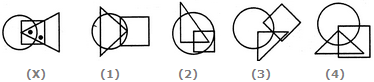# Non Verbal Reasoning - Dot Situation - Discussion

### Discussion :: Dot Situation - Section 1 (Q.No.15)

From amongst the figures marked (1), (2), (3) and (4), select the figure which satisfies the same conditions of placement of the dots as in figure (X).

15.

Select the figure which satisfies the same conditions of placement of the dots as in Figure-X.[A]. 1 [B]. 2 [C]. 3 [D]. 4

Explanation:

In fig. (X), one of the dots lies in the region common to the square and the triangle only and the other dot lies in the region common to all the three figures - the circle, the square and the triangle. In each of the alternatives (1) and (2), there is no region common to the square and the triangle only. In alternative (3), there is no region common to all the three figures. Only, alternative (4) consists of both the types of regions.